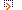# Scientific DirectoryFeatured Listings

math.furman.edu: Mathematical Quotation Server
Only mathematical quotations.
Keywords: math , math quotations , math quotes , mathematical , mathematical quotes
math.furman.edu/~mwoodard/mqs/mquot.shtml

Quotations against the intense dullness in today's software practice.

Site Listings

math.furman.edu: Mathematical Quotation Server
Only mathematical quotations.
Keywords: math , math quotations , math quotes , mathematical , mathematical quotes
math.furman.edu/~mwoodard/mqs/mquot.shtml